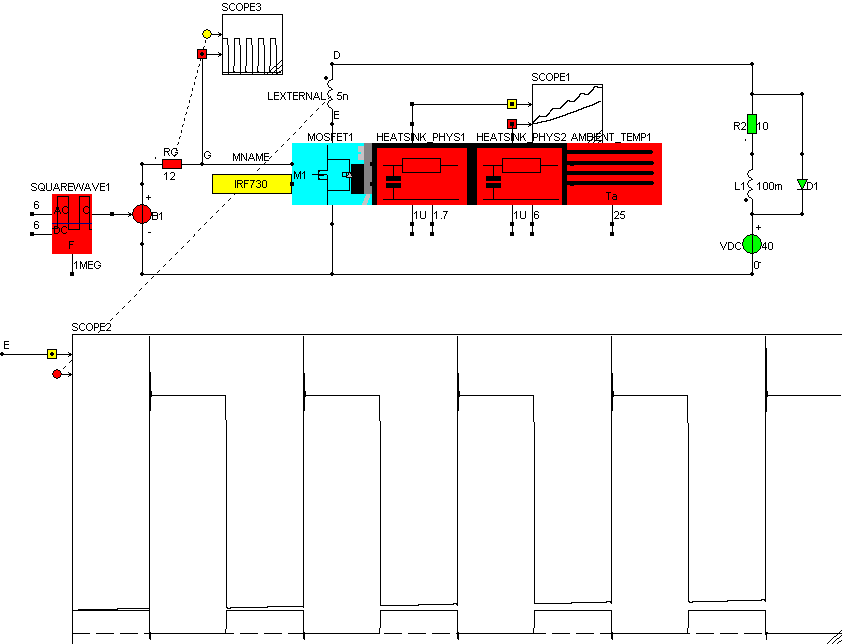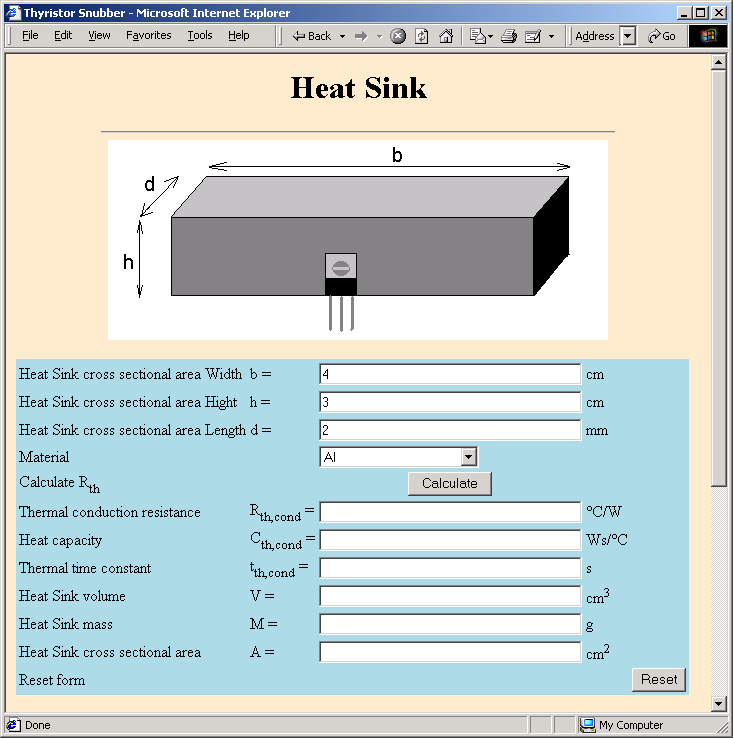• Introduction
• What is in this manual
• What is Caspoc
• User interface
• Introduction
• Starting
• Simulation
• Editing
• Viewing and printing
• Getting Started
• Basic editing
• Simulation in the time domain
• Basic User Interface Topics
• Editing
• Simulation
• Viewing
• Library
• Reports
• Project management
• Circuit and Block Diagram Components
• Introduction
• Cscript and user defined functions
• Component parameters
• Modeling Topics
• Introduction
• Power Electronics
• Semiconductors
• Electrical Machines
• Electrical drives
• Power Systems
• Mechanical Systems
• Thermal Systems
• Magnetic Circuits
• Green Energy
• Coupling to FEM
• Experimenter
• Analog hardware description language
• Embedded C code Export
• Coupling to Spice
• Small Signal Analysis
• Matlab coupling
• Tips and tricks
• Appendices

## Heatsink modeling.

All the dynamic semicondutor models from the library have on the right side a connection for a heatsink model.

The upper connection represents the losses in the model, the lower connection represents the temeprature at the case.

Select a heatsink model from the library heatsink and connect it to the right side of the semiconductor model. More heatsink models can be placed after each other, but at the end, a block "Ambient" from the library heatsink has to be placed.For each heatsink model Rth and Cth have to be defined, where Rth is the thermal resistance (C/W) and Cth (Ws/C) models the heat transport delay Cth=tdelay/Rth

The values for Rth and Cth can be calculated using the tool Heatsink from the tools section in Caspoc.